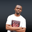Related Tags

euphoria
maths
communitycreator

# How to get a list of prime numbers in EuphoriaNDUKWE CHIDERA K.

### Overview

Prime numbers are numbers that are only divisible by one and themselves. We use the prime_list() function to get a list of prime numbers in Euphoria.

### What is prime_list()?

This function is built into the prime.e package of Euphoria’s standard library. This built-in function makes a comprehensive list of all the prime numbers which are less or equal in value to the limit indicated. With this function, we can only specify the upper limit of prime numbers to be returned since the lower limit is automatically considered as 1.

### Syntax

prime_list(limit)


### Parameter

• limit: This is the largest value that can be returned in the list of the prime numbers. Simply put, all members of the list returned would be less than or equal to the limit.

For example, if we want to return the list of prime numbers not greater than 100, the limit parameter will be 100.

### Code

In the code snippet below, the prime numbers between different ranges are returned:

include std/primes.e

puts(1,"These are the prime numbers <=10 :  ")
print(1,prime_list(10))

puts(1,"\nThese are the prime numbers <=200 :  ")
print(1,prime_list(20))

puts(1,"\nThese are the prime numbers <=50 :  ")
print(1,prime_list(50))
Generating the prime numbers

### Explanation

• Line 1: We include the primes.e package from the standard library. This allows us to use the prime_list() function.
• Line 3, 6 & 9: Here, a simple comment is put out to display.
• Line 4, 7 & 10: In these lines, we use the prime_list() function to return prime numbers in the indicated range, and the output is printed to the screen.

RELATED TAGS

euphoria
maths
communitycreator

CONTRIBUTORNDUKWE CHIDERA K.
RELATED COURSES

View all Courses

Keep Exploring

Learn in-demand tech skills in half the time